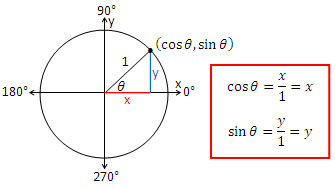# Trigonometry: The Unit CircleThis post is part of a series of posts on Trigonometry. To see all the posts, click on the tag #TrigonometryTutorials below. This is the post you should read before you read this.

So far we have only defined the trig functions on angles that are strictly less than 90 degrees. If you 'played' with your calculator you will notice that the calculator and calculate sines and cosines and tangents of angles larger than 90 degrees. Why does the calculator output "Math Error" when you try calculating the Tangent of 90? Why is Sine 90 degrees 1?

To answer this we need to Unit Circle. In a cartesian Co-ordinate plane, imagine a circle centred at the origin with radius 1. A line segment is drawn from the Origin to the boundaries of the circle, making an angle $\theta$ with the $x$-axis. Then, you drop a perpendicular down onto the $x$ axis. Given that $theta$ is between 0 degrees and 90 degrees, you basically have a normal right triangle! Let the point where the line segment intersects the circle be $(x,y)$. The Radius of the Circle is the hypotenuse, the opposite of $\theta$ is $y$ and the adjacent is $x$. Since the Hypotenuse is one, Using the Unit circle, we can redefine the trig ratios as:

$\sin \theta = y$ $\cos\theta = x$ $\tan\theta = \frac yx$

Now we have defined the Trig ratios for angles larger than 90, it is the $x$ and $y$ co-ordinate readings of the circle that creates the angle.

The is why the Trig functions are also sometimes called the Circular functions, because they can be defined by the co-ordinate readings on the circle

The Next post in this series is hereNote by Yan Yau Cheng
7 years, 5 months ago

This discussion board is a place to discuss our Daily Challenges and the math and science related to those challenges. Explanations are more than just a solution — they should explain the steps and thinking strategies that you used to obtain the solution. Comments should further the discussion of math and science.

When posting on Brilliant:

• Use the emojis to react to an explanation, whether you're congratulating a job well done , or just really confused .
• Ask specific questions about the challenge or the steps in somebody's explanation. Well-posed questions can add a lot to the discussion, but posting "I don't understand!" doesn't help anyone.
• Try to contribute something new to the discussion, whether it is an extension, generalization or other idea related to the challenge.
• Stay on topic — we're all here to learn more about math and science, not to hear about your favorite get-rich-quick scheme or current world events.

MarkdownAppears as
*italics* or _italics_ italics
**bold** or __bold__ bold
- bulleted- list
• bulleted
• list
1. numbered2. list
1. numbered
2. list
Note: you must add a full line of space before and after lists for them to show up correctly
paragraph 1paragraph 2

paragraph 1

paragraph 2

[example link](https://brilliant.org)example link
> This is a quote
This is a quote
    # I indented these lines
# 4 spaces, and now they show
# up as a code block.

print "hello world"
# I indented these lines
# 4 spaces, and now they show
# up as a code block.

print "hello world"
MathAppears as
Remember to wrap math in $$ ... $$ or $ ... $ to ensure proper formatting.
2 \times 3 $2 \times 3$
2^{34} $2^{34}$
a_{i-1} $a_{i-1}$
\frac{2}{3} $\frac{2}{3}$
\sqrt{2} $\sqrt{2}$
\sum_{i=1}^3 $\sum_{i=1}^3$
\sin \theta $\sin \theta$
\boxed{123} $\boxed{123}$

## Comments

There are no comments in this discussion.

×

Problem Loading...

Note Loading...

Set Loading...